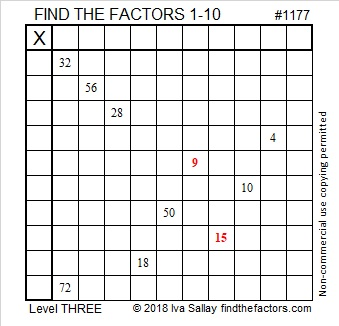# 1177 and Level 3

Why are two of the clues in today’s level three puzzle in red?

You still figure out the common factor of 32 and 72, then work down the first column cell by cell filling in factors as you go, BUT you won’t be able to know what factors to use for 9 unless you look at the number 15 first. You don’t have a problem with that, do you?Print the puzzles or type the solution in this excel file: 10-factors-1174-1186

Now I’ll tell you some facts about the number 1177:

• 1177 is a composite number.
• Prime factorization: 1177 = 11 × 107
• The exponents in the prime factorization are 1 and 1. Adding one to each and multiplying we get (1 + 1)(1 + 1) = 2 × 2 = 4. Therefore 1177 has exactly 4 factors.
• Factors of 1177: 1, 11, 107, 1177
• Factor pairs: 1177 = 1 × 1177 or 11 × 107
• 1177 has no square factors that allow its square root to be simplified. √1177 ≈ 34.307431177 is a palindrome in two bases:
It’s 414 in BASE 17 because 4(17²) + 1(17) + 4(1) = 1177
and 1E1 in BASE 28 (E is 14 base 10) because 28² + 14(28) + 1 = 1177

This site uses Akismet to reduce spam. Learn how your comment data is processed.